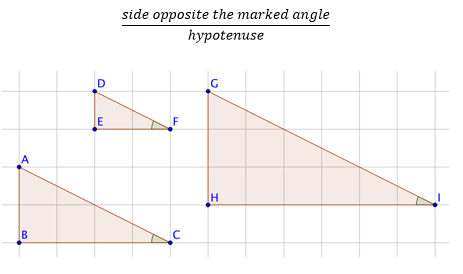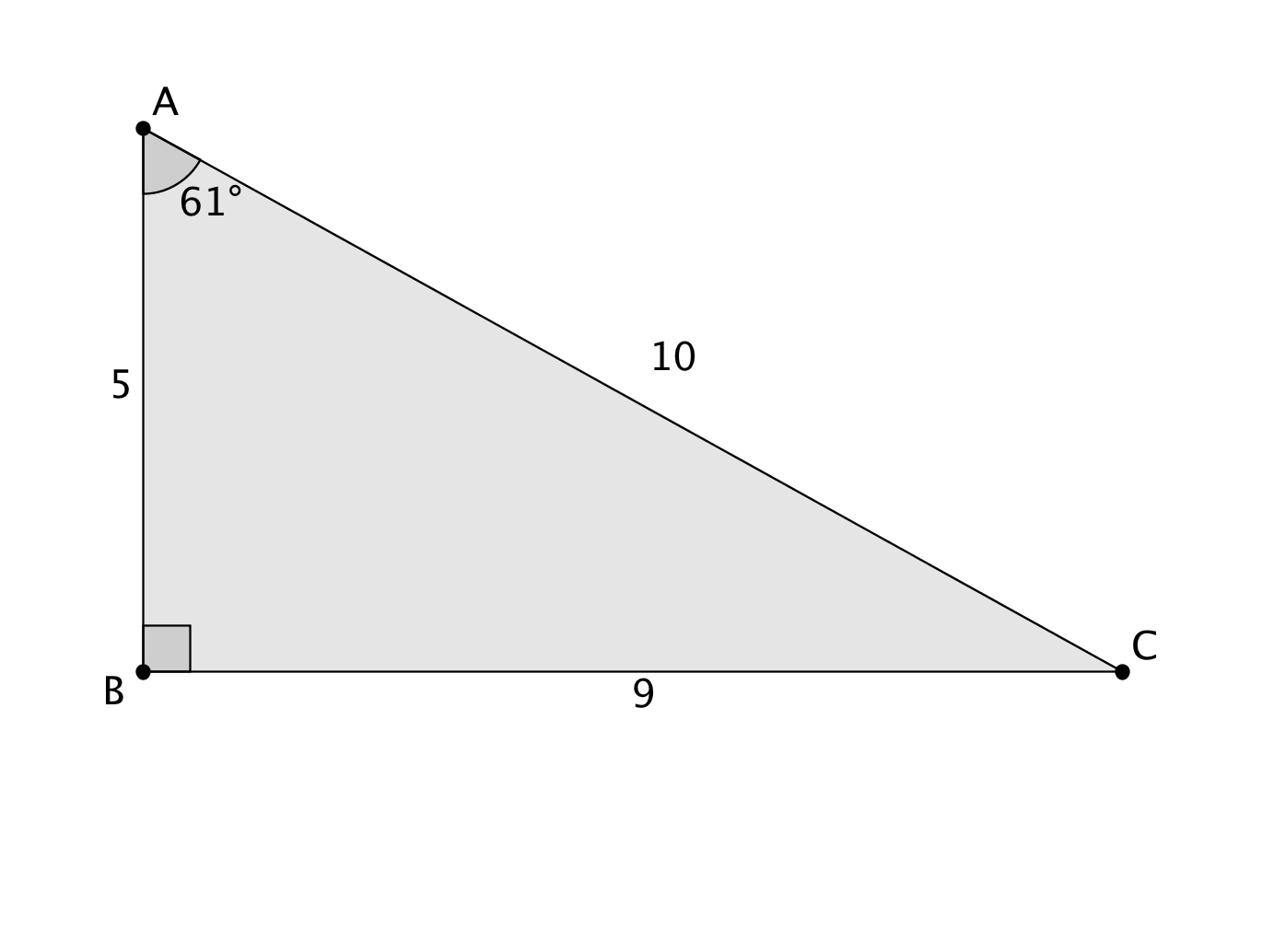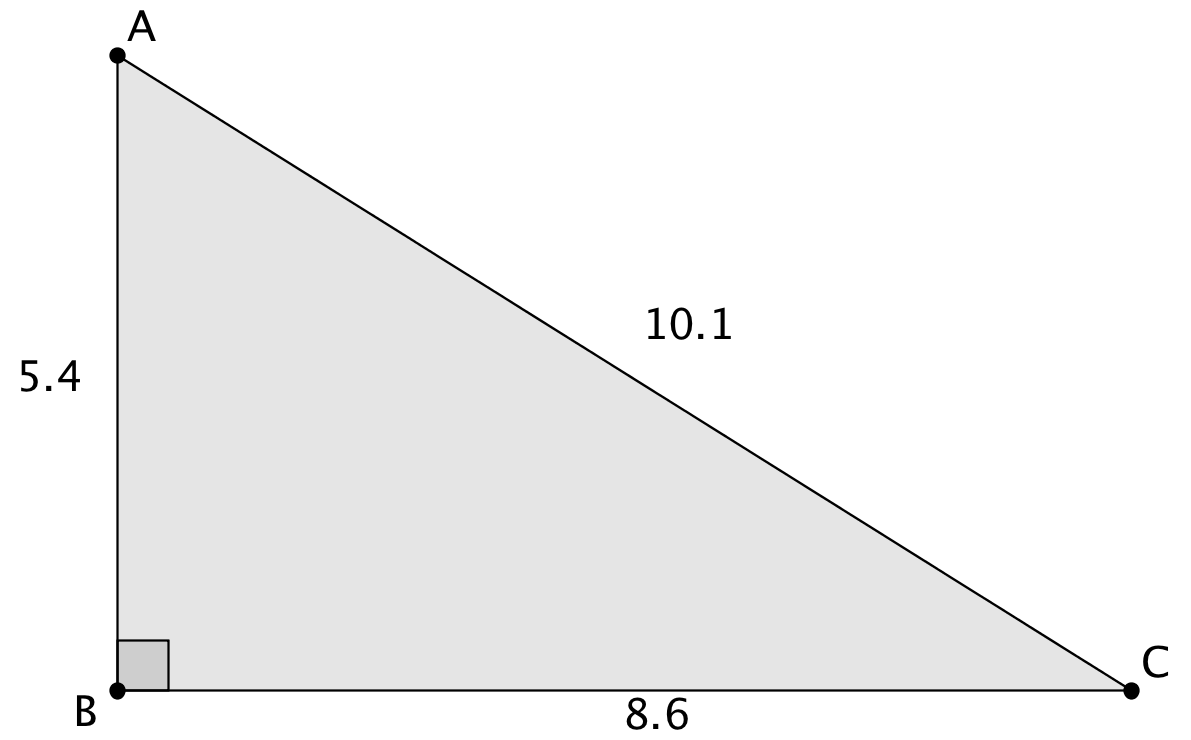# Right Triangles and Trigonometry

## Objective

Define and calculate the sine of angles in right triangles. Use similarity criteria to generalize the definition of sine to all angles of the same measure.

## Common Core Standards

### Core Standards

?

• G.SRT.C.6 — Understand that by similarity, side ratios in right triangles are properties of the angles in the triangle, leading to definitions of trigonometric ratios for acute angles.

?

• G.CO.C.10

## Criteria for Success

?

1. Calculate the ratio of the opposite side to the hypotenuse of a 30° angle in similar right triangles.
2. Define the sine of any given angle in a right triangle as the ratio of the side length of the opposite side to that angle over the hypotenuse (SOH).
3. Describe that the sine of any given angle is equal across all triangles with the same angle measures, extending from the angle-angle criterion for similarity.
4. Calculate the sine of any degree measure in a triangle using a scientific or graphing calculator.
5. Identify and memorize the sine for common angle measures of 0°, 30°, 45°, 60°, and 90°.

## Tips for Teachers

?

• Memorization of the sine for common angle measures is not required by the Common Core standards. However, if students have these values memorized, they will be better able to access some Algebra 2 and AP Calculus material.
• The following resource can help to frame the overall study of introductory trigonometry: Continuous Everywhere but Differentiable Nowhere, Sam Shah, “My Introduction to Trigonometry Unit for Geometry

## Anchor Problems

?

### Problem 1

Below is a set of similar right triangles. Find the ratio of the side lengths within each triangle that describe the side opposite the marked angle divided by the hypotenuse.### Problem 2

What is the sine of 0°, 45°, 60°, and 90°?

### Problem 3

${\triangle ABC}$ is a right triangle. What is the sine of ${\angle BAC}$?## Problem Set

?

The following resources include problems and activities aligned to the objective of the lesson that can be used to create your own problem set.

• Include problems where students need to identify the sine of angle measures that are not benchmark angle measures and then compare them to benchmark measures.
• Include problems where students need to identify similar triangles based on the sine.
• Include problems where the sine and one side are given and students need to find the value of the other side. Extend this problem such that students then need to find the third side using the Pythagorean theorem.

?

### Problem 1

Explain how you know that the following statement is always, sometimes, or never true:

“You can find the sine of any angle in any triangle by finding the ratio of the length of the opposite side over the length of the hypotenuse.”

### Problem 2

Find the sine of each angle shown in the diagram below. Then, predict between which two benchmark angle measures the angles fall between based on the sine.